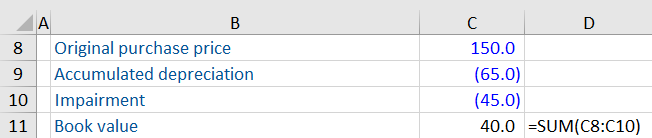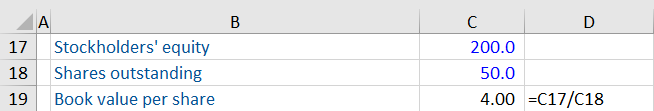## What is Book Value?

Book value represents the value of assets and liabilities at the date they are reported in a company’s documents. Book values are important for valuation purposes because they are based on accounting principles that are calculated consistently for all companies. For most assets and liabilities, book values are based on the historic cost of items.

As an accounting calculation, book value is different from an asset’s market value, which is contingent on supply and demand, and perceived value.

## Key Learning Points

• Book value is the value of assets and liabilities at the date they are reported in the company’s financial statements
• The book value does not represent the market value of the asset or liability and these can vary significantly
• A company’s book value is calculated as its total assets minus its total liablities
• The book value of a tangible (physical) asset is the carrying value after accumulated depreciation and any impairment expenses
• Book value is particularly useful when analyzing industries whose operations are based on their asset value (banks, precious metals, oil and gas)

## Tangible Assets

An asset’s book value or carrying value on the balance sheet is determined by subtracting accumulated depreciation from the initial cost or purchase price of the asset. Depreciation represents the use of an asset over its useful economic life.

Book Value of an Asset Formula

Book Value of an Asset = Purchase price – Accumulated Depreciation – Impairment

### Example

Company A bought a machine for \$150,000. Accumulated depreciation of \$65,000 has been charged to the machine as well as \$45,000 in impairment charges. Calculate the asset’s book value.All figures are in (\$) thousands

## Net Book Value of a Company

The net book value of a company shows the total book value of all its assets and liabilities. The formula is shown below:

### Net Book Value Formula

Book Value = Total Assets – Total Liabilities

Thus, net book value is calculated by taking the book value of a company’s non-current assets (including land, buildings, computers, etc.), plus the book value of a company’s current assets (including inventory, cash etc) and subtracting the book value of a company’s current and non-current liabilities (such as accounts payable and debt).

The net book value of a company is not the same as the market value of a company, since the book values of the assets and liabilities are not the same as the market values of all the assets and liabilities. However, it does provide an important function for users of accounts since it is based on prudent principles, and can sometimes be used to indicate the minimum value (or floor value) that the company is worth.

## Book Value per Share

Is a ratio that compares the net book value of a company with its shares outstanding. In other words, the book value of equity divided by the number of shares issued. It is calculated as:

Book Value per Share Formula

Book Value per Share = Shareholders’ Equity / Common Shares Outstanding

### Example

Company B has stockholders’ equity of \$200,000 and 50,000 shares outstanding. Calculate the book value per share.